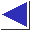# comp.lang.c FAQ list ·Question 20.12

Q: What is the most efficient way to count the number of bits which are set in an integer?

A: Many ``bit-fiddling'' problems like this one can be sped up and streamlined using lookup tables (but see question 20.13). Here is a little function which computes the number of bits in a value, 4 bits at a time:

```static int bitcounts[] =
{0, 1, 1, 2, 1, 2, 2, 3, 1, 2, 2, 3, 2, 3, 3, 4};

int bitcount(unsigned int u)
{
int n = 0;

for(; u != 0; u >>= 4)
n += bitcounts[u & 0x0f];

return n;
}
```